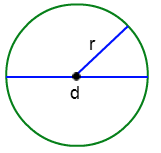Radius formula is simply derived by halving the diameter of the circle. When we connect a point on the circumference of a circle to the exact centre, then the line segment made is called the radius of the ring. The distance between the center of the circle to its circumference is the radius. The diameter is always double the radius. Hence, the formula is derived by dividing the diameter by 2.The Radius Formula in terms of diameter, circumference, and the area is given as,

Radius in Terms of Diameter d ⁄ 2
Radius in Terms of Circumference C ⁄ 2π
Radius in Terms of Area √(A ⁄ π)

Where,

• r is the radius of the circle
• d is the diameter of the circle
• C is the circumference of the circle
• A is the area of the circle

### Solved Example Questions

Question 1: Find the radius of the circle whose diameter is 19 cm?

Solution:

Given,

Diameter of the circle = d = 19 cm

Radius of the circle = (d ⁄ 2) = 19 ⁄ 2 cm = 9.2 cm

Question 2: Find the radius of the circle whose diameter is 30 cm?

Solution:

Given,

Diameter of the circle = d = 30 cm

Radius of the circle = (d ⁄ 2) = 30 ⁄ 2 cm = 15 cm

Question 3: If the area of a circle is 154 cm2, then find the radius of the circle.

Solution:
Given,
Area of the circle = A = 154 cm2
Let “r” be the radius of the circle.

$$\begin{array}{l}=\sqrt{\frac{154}{\frac{22}{7}}}\\ =\sqrt{\frac{154\times 7}{22}}\\=\sqrt{7\times 7}\\=7\end{array}$$

Hence, the radius of the circle = 7 cm.

Question 4: Find the radius of the circle whose circumference is 22 cm.

Solution:
Given,
Circumference of the circle = C = 22 cm
Let “r” be the radius of the circle.
$$\begin{array}{l}=\frac{22}{2\times \frac{22}{7}}\\=\frac{7}{2}\\=3.5\end{array}$$